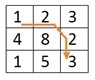# Min Cost Path

A matrix of the different cost is given. Also, the destination cell is provided. We have to find minimum cost path to reach the destination cell from the starting cell (0, 0).

Each cell of the matrix represents the cost to traverse through that cell.

From a cell, we cannot move anywhere, we can move either to the right or to the bottom or to the lower right diagonal cell, to reach the destination.

## Input and Output

Input:
The cost matrix. And the destination point. In this case the destination point is (2, 2).
1 2 3
4 8 2
1 5 3

Output:
The minimum cost to reach to the destination from (0, 0). The minimum cost is 8.## Algorithm

minCostPath(destX, destY, cost)

Input − The (x, y) place of the destination, and the cost matrix.

Output − Minimum cost to reach a destination.

Begin
define matrix totalCost, whose order is same as cost matrix
totalCost[0, 0] = cost[0, 0]

for i := 1 to destX, do
totalCost[i, 0] := totalCost[i-1, 0] + cost[i, 0]
done

for j := 1 to destY, do
totalCost[0, j] := totalCost[0, j-1] + cost[0, j]
done

for all places (i, j) from (1, 1) to (destX, destY), do
totalCost[i, j] := minimum of totalCost[i-1, j-1], totalCost[i-1, j] and (totalCost[i, j-1] + cost[i,j])
done

End

## Example

#include<iostream>
#define ROW 3
#define COL 3
using namespace std;

int cost[ROW][COL] = {
{1, 2, 3},
{4, 8, 2},
{1, 5, 3}
};

int min(int a, int b, int c) {
return (a<b)?((a<c)?a:c):((b<c)?b:c);
}

int minCostPath(int destX, int destY) {
int totalCost[ROW][COL];

totalCost = cost;

for (int i = 1; i <= destX; i++)
totalCost[i] = totalCost[i-1] + cost[i];    //set first col of totalCost array

for (int j = 1; j <= destY; j++)            //set first row of totalCost array
totalCost[j] = totalCost[j-1] + cost[j];

for (int i = 1; i <= destX; i++)            //for second row and column to all
for (int j = 1; j <= destY; j++)
totalCost[i][j] = min(totalCost[i-1][j-1], totalCost[i- 1][j], totalCost[i][j-1]) + cost[i][j];
}
Minimum Cost: 8